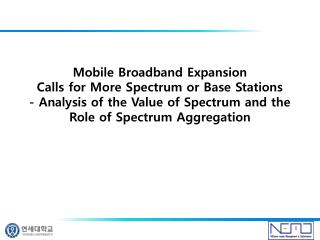Download PresentationContents

# Contents

Download Presentation## Contents

- - - - - - - - - - - - - - - - - - - - - - - - - - - E N D - - - - - - - - - - - - - - - - - - - - - - - - - - -
##### Presentation Transcript

1. Mobile Broadband ExpansionCalls for More Spectrum or Base Stations- Analysis of the Value of Spectrum and the Role of Spectrum Aggregation

2. Contents • Introduction • Methodology, models and assumptions • Analysis of engineering value • Analysis of Strategic value • Conclusions

4. Introduction • Analysis of the value of spectrum • Engineering value • strategic value • Addresses two research questions • How is the production cost affected by different levels of spectrums? • How does the use of spectrums aggregation influence the evaluation of spectrums? • The role and value of spectrum aggregation • modeling different operators with different amount of spectrums and cooperation strategies

5. Methodology, models and assumptions • The role and value of spectrum aggregation is carried out by modeling four different operators • Engineering value : cost savings in the infrastructure of an operator’s network • Strategic value : expected position and competitive advantage as a result of the assigned • Market and demand • By modeling an Urban area where four operators each has a 25% market share • 5, 20 and 80 GB per user and month. over eight hours per day, translating into a busy hour rate of 12.5%

6. Methodology, models and assumptions • Network modeling, coverage and capacity and data rates Capacity = Bandwidth (MHz) * number of sites * sectors * spectrum efficiency • Cost assumptions • Opex (Operational expenditures) is set to 20% of accumulated capex, in line with estimates made by Ofcom(2009) • cost of capital (WACC) is set to 12.9%.

7. Analysis of engineering value • Four operators with different spectrum competing on one market, network costs • How different levels of spectrum affect network costs • To capture the implicit value of spectrum as a function of lower network cost through different levels of spectrum. • Network Analysis • The four operators with similar networks, equipped with the same number of sites, each having a 25% market share • The amount of spectrum allocated is different (from 10 MHz, 20 MHz, 40 MHz to 80 MHz applicable for LTE services)

8. Analysis of engineering value Figure2:Utilization rate of the four network Figure1:Spectrum,peak rate and capacity per km2 for the four operator Figure4: Capex to upgrade Figure3:Number of sites required to manage increased traffic

9. Analysis of engineering value

10. Analysis of engineering value • Conclusions engineering value • Operators with less amount of spectrum have options to provide mobile broadband services. • Aradical assumption of usage in order to stretch the analysis and show the impact of increase usage to levels that currently looks unlikely. • Operators that are able to obtain more spectrum than its competition, and also pursue network sharing, and spectrum aggregation

11. Analysis of Strategic value • How the value of spectrum can be influenced by the user perception of service quality in terms of offered data rates. • Offered data rates • Operators with more bandwidth are able to claim that “we can offer higher data rates” • Higher peak data rates can be provided • More users can be served at a given data rate when the network is loaded

12. Analysis of Strategic value • Impact of carrier aggregation and network modeling Difference of carrier aggregation

13. Analysis of Strategic value • Impact of carrier aggregation and network modeling User satisfaction depends on user expectation

14. Analysis of Strategic value • Discussion • Two conclusions about user expectations and user satisfaction • It is important to provide what is promised • User will notice and compare if other operators can provide “more”

15. Conclusions • Operator with access to 80 MHz is able to lower its production cost with 94%when the usage increase from 5 to 80 GB. • There is a direct relation between the amount of spectrum and production cost • The competition on spectrum is set to increase, and spectrum aggregation is set to play a key role on the market • Contributions • Consider both the engineering and strategic value • Take into account existing infrastructure and consider both upgrading of existing sites and deployment of new sites